# Examples of geospatial SQL (I)BBVA API Market

In a previous post, we presented a brief introduction to geospatial SQL, mentioning the Dimensionally Extended nine-Intersection Model (DE-9IM), how it is implemented via spatial predicates and the concept of geospatial index. In this and the following post we will be looking at some specific examples of geospatial SQL queries.

In these two posts, we will be using test data that correspond to Colombia and can be downloaded here. After unzipping the file, you can see that it contains four CSV files.You can load these data directly into CartoDB directly to do the exercises, thereby avoiding having to install any other software in your computer. Since CartoDB is based on PostreSQL and the spatial extension PostGIS, you can use the SQL API of PostGIS

To load data in CartoDB, the first step is to sign up in the platform. After doing so, authenticate and go directly to the Dashboard.

To load the data file, simply drag it to the Dashboard and CartoDB will automatically create a table in PostreSQL and take you to a screen that displays the data. A screenshot of the barrios_of_bogota table can be seen in the following image. We will be using this extensively in the examples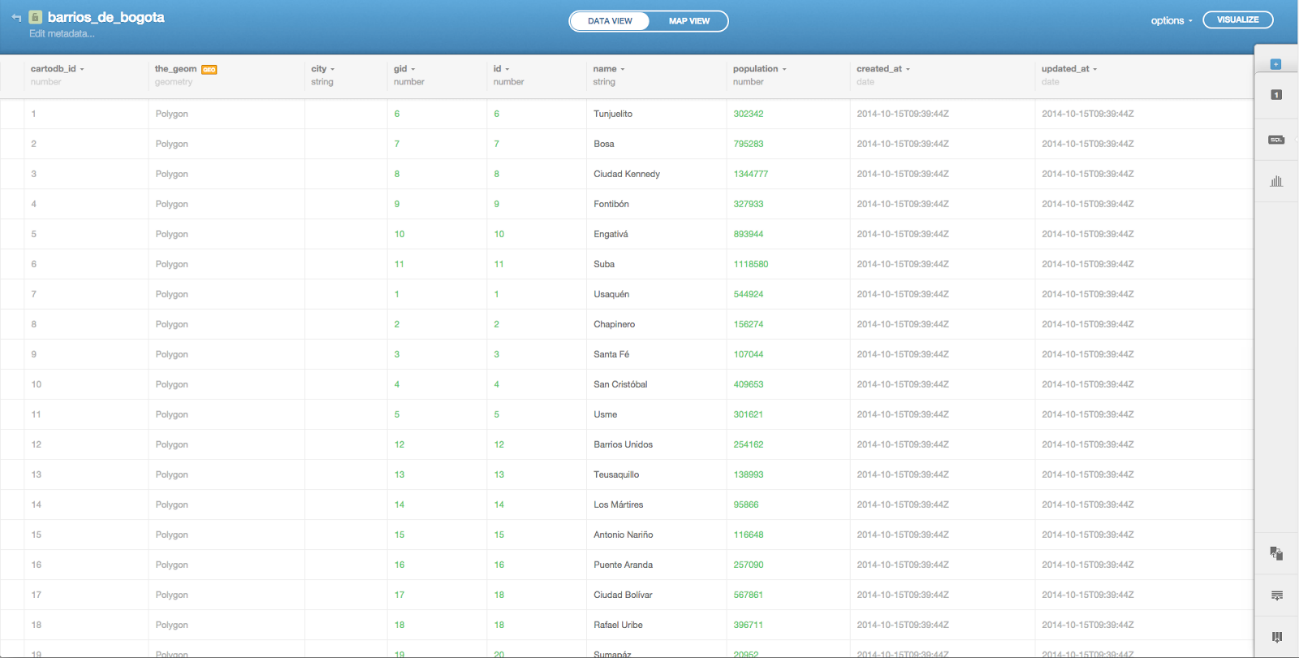Click on Map View at the top to see the data directly on a map.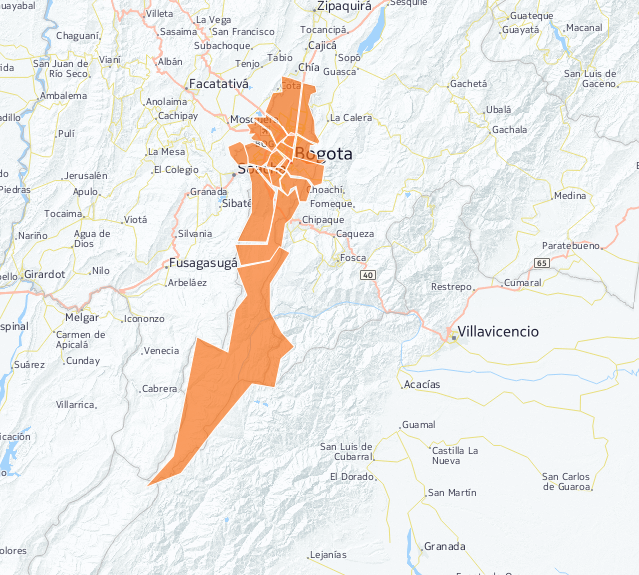In addition, In addition, spatial indexes have already been generated on the geometric column, so we are now ready to start running queries using these data.

Repeat the process with the rest of the files, after which you are ready to start working., so we are now ready to start running queries using these data.

Use of simple geospatial predicates

The next step, deals with the practical use of the geospatial predicates mentioned in the previous post and how they are applied to the specific data that have been loaded in CartoDB

Intersects

We are going to perform a test with the ST_Intersects predicate in the barrios_de_bogota table, made up of polygons. To do so, the first step is to label the polygons with their ID so they can be referenced in the queries.

Labeling polygons in CartoDB is very easy. Simply follow these steps

In the map display of the table, click on the wizards icon on the side barIn the first wizard (simple), select In the first wizard (simple), select gid in the pull-down Label Text. Choose a font size that is large enough to be seen clearly (18, in the example) in the pull-down Label Text. Choose a font size that is large enough to be seen clearly (18, in the example)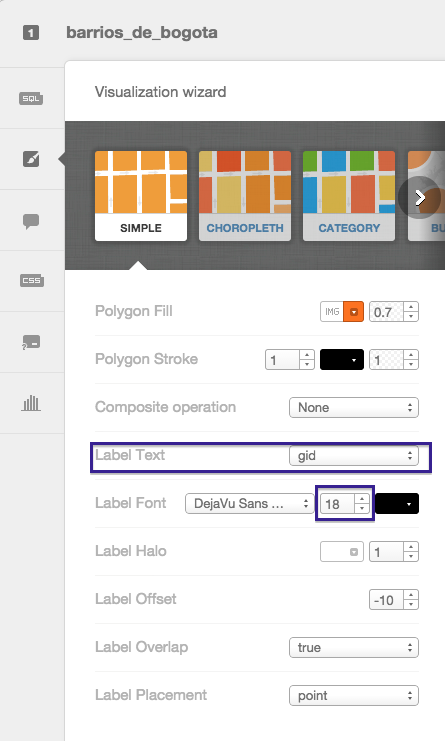You can now see the labeled polygons with their gid attribute.

As shown in the screenshot, the polygons that represent the barrios of Bogotá intersect each other. Specifically, note that the polygon with the 16 gid intersects with the polygons containing gids 8 and 15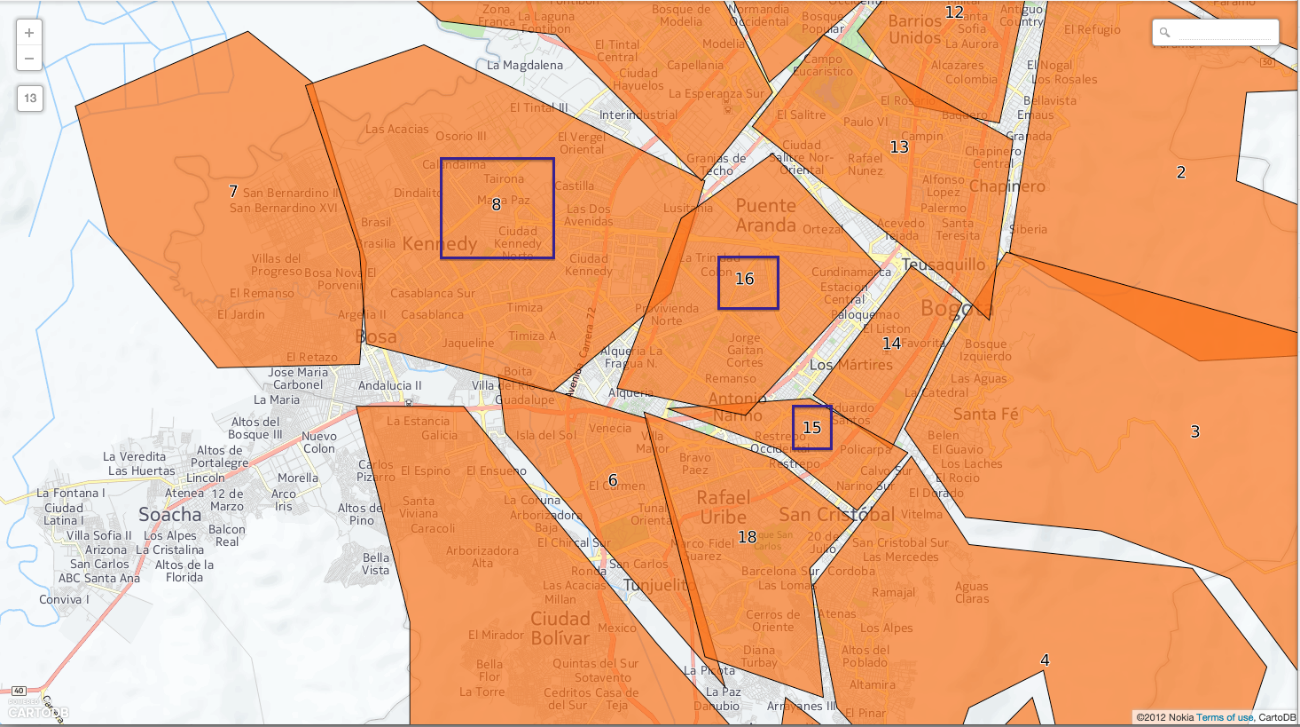You can verify this with a geospatial SQL query that can be run in the CartoDB SQL consoleWITH poligono_16 as (select the_geom from barrios_de_bogota where gid = 16)

SELECT gid
FROM barrios_de_bogota
WHERE ST_Intersects(the_geom, poligono_16.the_geom)
AND gid != 16

As expected, the result is gids 8 and 15.There are two details that should be highlighted as regards this query:

By definition, a polygon intersects with itself. Therefore the condition gid != 16 is added to exclude the polygon itself from the comparison

By using the WITH clause, you avoid having to run the query several times by precalculating the main query and using the result again in the same query.  The alternative query would have been as follows

SELECT gid
FROM barrios_de_bogota
WHERE ST_Intersects(the_geom, (select the_geom from barrios_de_bogota where gid = 16))
AND gid != 16

Note that this second query runs the most internal SELECT instruction once per each row in the barrios_de_bogota table. Which is not necessary. In this case, since the table is rather small, you will not notice much difference in processing times. However, in large tables that contain millions of records, optimized queries like this one can mean the difference between obtaining a result in a reasonable period of time or not.

Contains

The next step is an example of the Contains predicate, to answer the question What barrio is the Museo del 20 de Julio in?

Run the query again from the SQL console of the barrios_de_bogota table

SELECT b.name from barrios_de_bogota b, points p
WHERE ST_Contains(b.the_geom, p.the_geom) and
p.name = 'Museo del 20 de Julio'

The result is San Cristobal, as expected.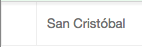Two tables were used in this query. The barrios_de_bogota table and the points table, which contains the POIs (points of interest) of Colombia, one of which is the Museo del 20 de Julio

Distance

This section will deal with one of the most common uses: distance calculation. With a small variant. We will apply the ST_DWithin predicate, implemented by PostGIS, to calculate the geometric elements at a maximum distance from a given point.

Suppose you want to know which points of interest are within a maximum distance of 2 km from the Office of Tourism bogotanismo.com, in the city of Bogotá. We can do so by running the following SQL query

WITH oficina_turismo as (SELECT ST_Transform(the_geom, 21818) as the_geom

FROM points

WHERE name='Bogotanisimo.com')

SELECT name

FROM points a, oficina_turismo b

WHERE

name is not null and

name != '' and

name != 'Bogotanisimo.com' and

ST_DWithin(

ST_Transform(a.the_geom, 21818),

b.the_geom,

2000

)

The result of the query is the Los Hornitos bakery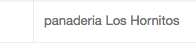The WITH clause has also been used in this query to avoid repeating queries. We also used the ST_DWithin predicate to calculate the points at a maximum distance from the point we were interested in. Lastly, note the use of the ST_Transform function of PostGIS. The purpose of this function is to transform the geometric elements from one system of coordinates to another. This is necessary because the geometric elements stored in the tables use a geographic system of coordinates, where the units of measurement are degrees, and not meters. Therefore, to measure distances in meters, we have to use a system of coordinates that uses meters, such as the SRID 21818, used in Colombia.

Crosses

Finally, an example of how to use the ST_Crosses predicate of PostGIS to easily obtain the names of all the barrios in Bogotá crossed by the Bogotá River. Do so by running the following query from the SQL console of the barrios_de_bogota table

SELECT b.name
FROM barrios_de_bogota b JOIN waterways w
ON ST_Crosses(b.geom, w.geom)
WHERE w.name = 'Rio Bogotá'

The result is made up of several rows with the names of the barrios we were looking for.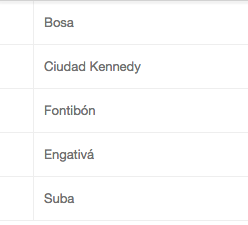This is the last spatial SQL exercise, for now. In the next post, we will see some simple examples of spatial analysis that use the spatial predicates provided by PostGIS.